KSEEB SSLC Class 10 Maths Solutions Chapter 13 Statistics Ex 13.1

KSEEB SSLC Class 10 Maths Solutions Chapter 13 Statistics Ex 13.1 are part of KSEEB SSLC Class 10 Maths Solutions. Here we have given Karnataka SSLC Class 10 Maths Solutions Chapter 13 Statistics Exercise 13.1.

Karnataka SSLC Class 10 Maths Solutions Chapter 13 Statistics Exercise 13.1

Question 1.
A survey was conducted by a group of students as a part of their environment awareness programme, in which they collected the following data regarding the number of plants in 20 houses in a locality. Find the mean number of plants per house.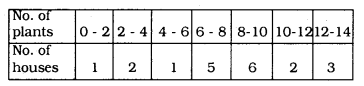Which method did you use for finding the mean, and why ?
Solution:
Average by Direct Method :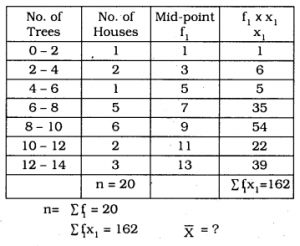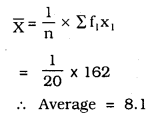Question 2.
Consider the following distribution of daily wages of 30 workers of a factory.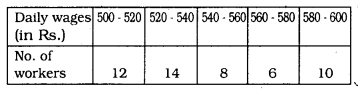Find the mean daily wages of the workers of the factory by using an appropriate method.
Solution:
Step deviation method :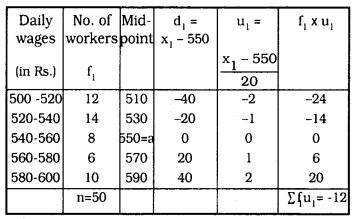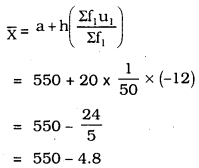∴ Average = 545.20.

Question 3.
The following distribution shows the daily pocket allowance of children of a locality. The mean pocket allowance is Rs. 18. Find the missing frequency f.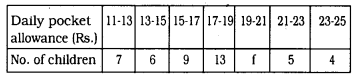Solution: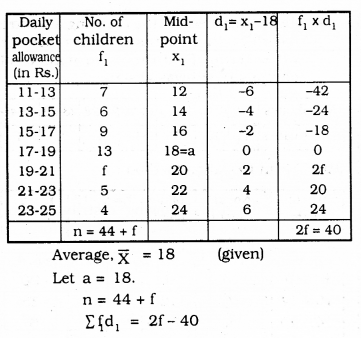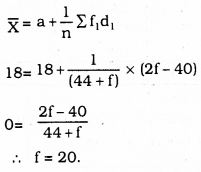Question 4.
Thirty women were examined in a hospital by a doctor and the number of heartbeats per minute were recorded and summarised as follows. Find the mean heartbeats per minute for these women, choosing a suitable method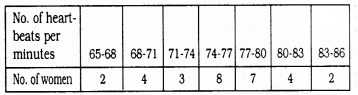Solution: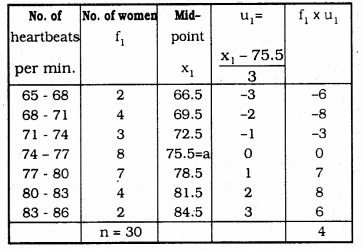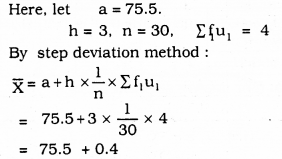∴ Mean heartbeats per minute = 75.9

Question 5.
In a retail market, fruit vendors were selling mangoes kept in packing boxes. These boxes contained varying number of mangoes. The following was the distribution of mangoes according to the number of boxes.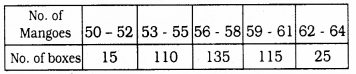Find the mean number of mangeos kept in a packing box. Which method of finding the mean did you choose ?
Solution:
Step Deviation Method :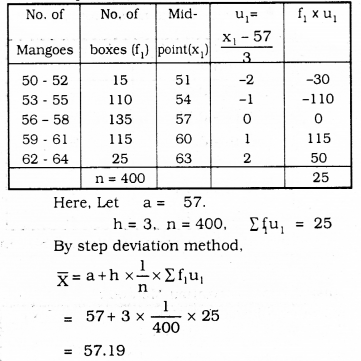∴ Mean Number of mangoes in the boxes = 57.19

Question 6.
The table below shows the daily expenditure on food of 25 households in a locality.
Find the mean daily expenditure on food by a suitable method.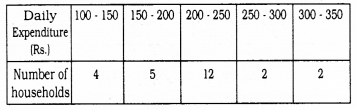Solution:
By Step deviation method: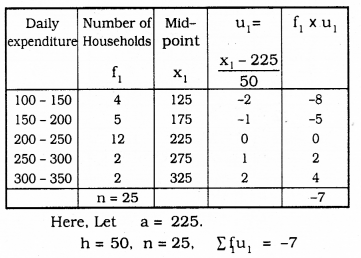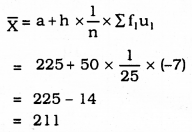∴ Mean Daily expenditure for food = Rs. 211.

Question 7.
To find out the concentration of SO2 in the air (in parts per million, i.e., ppm), the data was collected for 30 localities in a certain city and is presented below: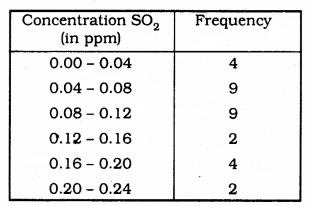Find the mean concentration of SO2 in the air.
Solution:
By step Deviation method: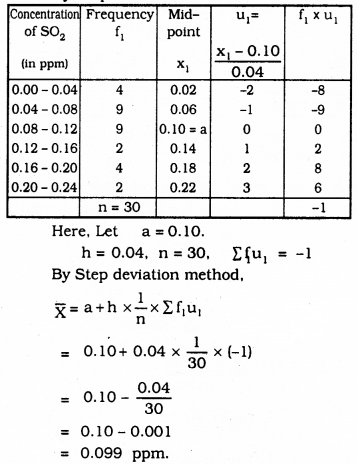∴ Mean Concentration of SO2 = 0.099 ppm.

Question 8.
A class teacher has the following absentee record of 40 students of a class for the whole term. Find the mean number of days a student was absent.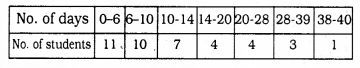Solution:
Mean Deviation method: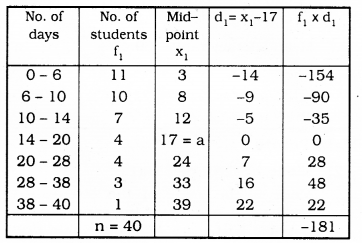Here, a = 17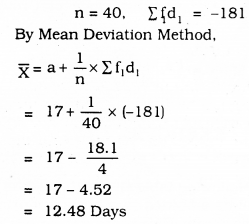∴ Mean number of days a student was absent = 12.48 Days.

Question 9.
The following table gives the literacy rate (in percentage) of 35 cities. Find the mean literacy rate.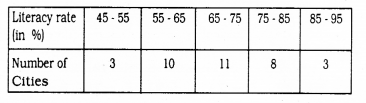Solution:
Step Deviation Method: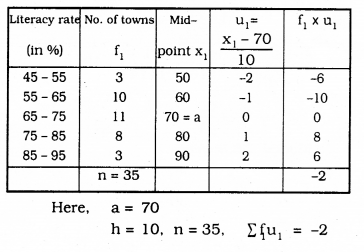By Step Deviation Method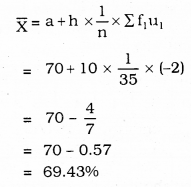∴ Mean Literacy rate = 69.43%

We hope the given KSEEB SSLC Class 10 Maths Solutions Chapter 13 Statistics Ex 13.1 will help you. If you have any query regarding Karnataka SSLC Class 10 Maths Solutions Chapter 13 Statistics Exercise 13.1, drop a comment below and we will get back to you at the earliest.